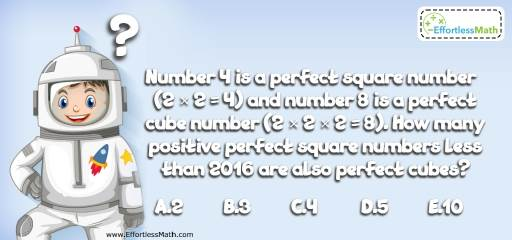# Number Properties Puzzle – Challenge 5

This is a great mathematics critical thinking challenge for those who love math puzzles and challenges. Let's see if you can solve this math puzzle!## Challenge:

Number 4 is a perfect square number (2 × 2 = 4) and number 8 is a perfect cube number (2 × 2 × 2 = 8). How many positive perfect square numbers less than 2016 are also perfect cubes?

A- 2

B- 3

C- 4

D- 5

E- 10

### The Absolute Best Book to challenge your Smart Student!

Because there aren’t that many perfect squares less than 2016 that is also a perfect cube, let’s look for the smallest perfect square number. This happens to be number 1. It is a perfect square number (1 × 1 = 1) and a perfect cube number (1×1×1=1). To find the next positive perfect square number less than 2015 that is also a perfect cube, let’s take a look at number 2. $$2^{2} = 4$$ is a perfect square and $$2^{3} = 8$$ is a perfect cube number and $$(2^{2})^{3} = 2^{6} = 64$$, which is both a perfect square and cube number. Next perfect square and cube number with the base of 2 is $$(2^{6})^{2} =2^{12} = 4096$$, which is greater than 2016.
With the same method, $$3^{6} = 729$$ is the next perfect square number and cube. $$4^{6} = 4096$$ is a perfect square and cube number, but is bigger than 2016. Therefore, numbers 1, 64 and 729 are the perfect square and cube numbers less than 2016.

### What people say about "Number Properties Puzzle – Challenge 5 - Effortless Math: We Help Students Learn to LOVE Mathematics"?

No one replied yet.

X
30% OFF

Limited time only!

Save Over 30%

SAVE $5 It was$16.99 now it is \$11.99